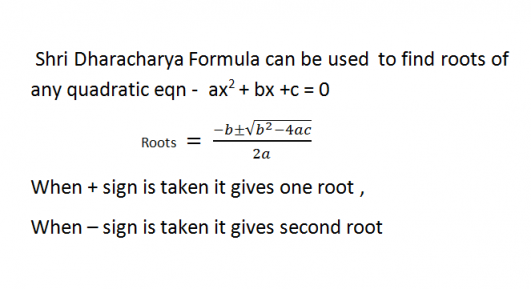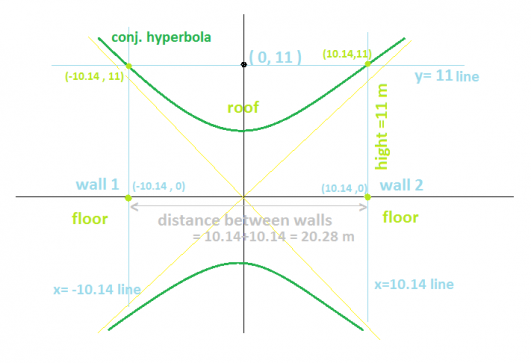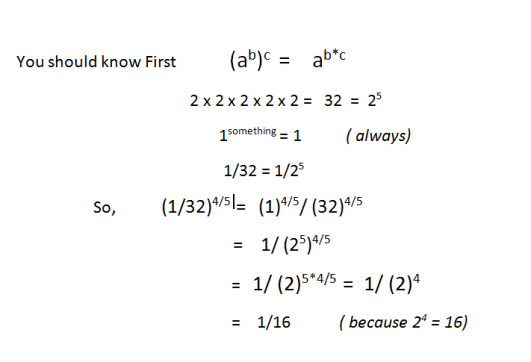if you know the roots then you can find their equation-

x^2 - (sum of roots) x + product of roots = 0

favorited this question.

Mean = sum of all values / no. Of values

I am also considering mean Anita's Age in Calculating Mean.

Let the age of Anita and others is a, b, c, d,e,f  respectively !

Now Mean of their age =(a + b + c + d + e + f) / 6 = 6 years

from here ,,    … Read more

favorited this question.
vikas sinah answered Jill Porras' question

true.

2 Line equations will have a unique solution if and only if they intersect each other at a particular point ( x, y ). If Lines are parallel, then they'll never intersect and hence we can not find any solution.

if we consider two parallel Lines of equations,say-

2x + 3y = 7 ,  2x + … Read more

favorited this question.
vikas sinah answered ly fen chen's question

Who knows what will happen in future ? It is just start of 20th century. Some people consider this century as no-war century while some don't.

we can not stop wars completely. Those who live in peaceful regions usually don't believe that there can be a big war in present time in any part of … Read more

favorited this question.y^2-x^2 = 18
if you look at the graph of this hyperbola
you just need to get the x coordinates where the line y= 11 ( hight )
is intersecting this hyperbolic curve.

To get the … Read more